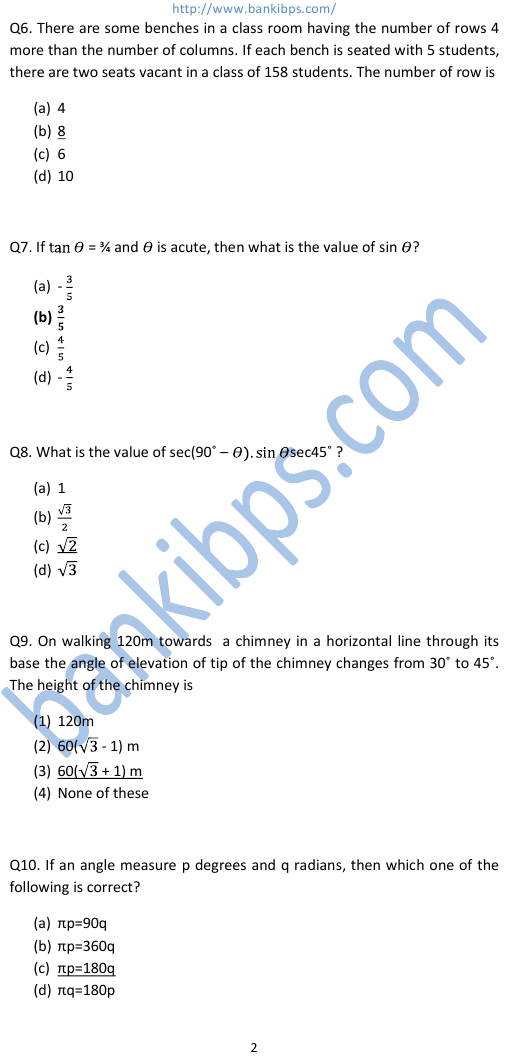# CDS Exam Question – CDS Exam Preparation Question Answer

Q1. What is the value of k will make the expression 4k2 + 12k + k a perfect square? 5 7 8 9 cds exam question Q2. If one root of the equation x^2/a + x/b + 1/c = 0 is reciprocal of the other, then which one of the following is correct? a=b b=c ac=1 a=c Q3. If f(x) is a polynomial with constant term 10 having a factor (x-k) where k is an integer, then what is the possible value of k? -20 20 8 5
Aptitude